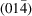International Tables for Crystallography (2019). Vol. H. ch. 4.8, pp. 473-488https://doi.org/10.1107/97809553602060000963

## Contents

• 4.8. Application of the maximum-entropy method to powder-diffraction data  (pp. 473-488)
• 4.8.1. Introduction  (pp. 473-474) | html | pdf |
• 4.8.2. The principle of maximum entropy  (pp. 474-475) | html | pdf |
• 4.8.3. Prior densities in MEM calculations  (pp. 475-476) | html | pdf |
• 4.8.4. Crystallographic MEM equations  (p. 476) | html | pdf |
• 4.8.5. MEM algorithms  (pp. 476-477) | html | pdf |
• 4.8.5.1. Exponential modelling algorithm (Gull & Daniell, 1978; Collins, 1982; Collins & Mahar, 1983)  (pp. 476-477) | html | pdf |
• 4.8.5.2. Sakata–Sato algorithm (Sakata & Sato, 1990; Sakata et al., 1990, 1992, 1993; Kumazawa et al., 1995)  (p. 477) | html | pdf |
• 4.8.5.3. Cambridge algorithm (Bryan & Skilling, 1980, 1986; Burch et al., 1983; Skilling & Bryan, 1984; Gull & Skilling, 1987, 1999)  (p. 477) | html | pdf |
• 4.8.5.4. Two-channel MaxEnt method (Papoular & Gillon, 1990; Zheludev et al., 1995; Papoular et al., 1996)  (p. 477) | html | pdf |
• 4.8.5.5. Valence-only MaxEnt method (Roversi et al., 1998)  (p. 477) | html | pdf |
• 4.8.6. Constraints in the MEM based on the measured intensities from powder-diffraction data  (pp. 477-480) | html | pdf |
• 4.8.6.1. F and G constraints  (pp. 478-479) | html | pdf |
• 4.8.6.2. Constraints using `partly phased' reflections for anomalous-scattering X-ray powder diffraction  (pp. 479-480) | html | pdf |
• 4.8.6.3. Prior-derived F constraints  (p. 480) | html | pdf |
• 4.8.6.4. Observed structure factors  (p. 480) | html | pdf |
• 4.8.7. Analysis of the electron-density distribution from powder-diffraction data  (pp. 480-485) | html | pdf |
• 4.8.7.1. Types of MEM-reconstructed electron-density distribution  (pp. 480-482) | html | pdf |
• 4.8.7.2. MEM-based improvement of the structure model from charge flipping  (p. 482) | html | pdf |
• 4.8.7.3. Combination of MEM and Rietveld refinement (REMEDY cycle)  (pp. 482-483) | html | pdf |
• 4.8.7.4. MEM-assisted structure solution by charge flipping  (pp. 483-484) | html | pdf |
• 4.8.7.5. Disorder and anharmonic atomic displacements  (p. 484) | html | pdf |
• 4.8.7.6. Accurate electron densities  (pp. 484-485) | html | pdf |
• 4.8.8. Extensions of the MEM  (pp. 485-486) | html | pdf |
• 4.8.8.1. Distribution of normalized residuals of the structure factors  (p. 485) | html | pdf |
• 4.8.8.2. Stopping criterion  (pp. 485-486) | html | pdf |
• 4.8.8.3. Generalization of MaxEnt algorithm to n dimensions (n > 3) and application to powder-diffraction data of aperiodic structures  (p. 486) | html | pdf |
• References | html | pdf |
• Figures
• Fig. 4.8.1. Single-crystal (left) and powder (right) X-ray diffraction data for Pb3O4  (p. 473) | html | pdf |
• Fig. 4.8.2. Electron-density distribution for threefold disordered pentamethyl­cyclopentadienyllithium (Li[C10H15], LiCp*)  (p. 474) | html | pdf |
• Fig. 4.8.3. Electron-density distribution in the (110) plane of Si, calculated from powder X-ray diffraction data  (p. 474) | html | pdf |
• Fig. 4.8.4. Dividing of the total intensity of the overlapping reflections into the contributions of individual reflections by Rietveld refinement (with a structural model) and by Le Bail fitting (without a structural model) for powder-diffraction data for K2C2O4 (adapted from Samy et al  (p. 478) | html | pdf |
• Fig. 4.8.5. Observed intensity (points) as a function of the scattering angle, together with lines representing (a) the calculated intensity of the Rietveld refinement and (b) the intensities after decomposition according to equation (4.8.22) (from Takata, 2008)  (p. 479) | html | pdf |
• Fig. 4.8.6. Flow chart of the procedure for reconstruction of the different MEM maps from powder-diffraction data  (p. 481) | html | pdf |
• Fig. 4.8.7. Structural model from Rietveld refinement and MEM electron densities of disordered α-Rb2C2O4 (left) and α-Rb2CO3 (right)  (p. 482) | html | pdf |
• Fig. 4.8.8. Flow chart of MEM/Rietveld method with structure refinement and MEM imaging of the charge density (adapted from Takata, 2008)  (p. 483) | html | pdf |
• Fig. 4.8.9. Acetylene adsorption isotherm and crystal structures of CPL-1 with adsorption of acetylene corresponding to the amount of adsorbed gas  (p. 483) | html | pdf |
• Fig. 4.8.10. The top panel shows the (110) plane of the crystal structure of SiAl4O2N4; red and yellow indicate fractional occupancies by oxygen and nitrogen, respectively; dark blue indicates the fractional occupancy of the (Si,Al)2A and (Si,Al)2B sites  (p. 484) | html | pdf |
• Fig. 4.8.11. Crystal structure and MEM-reconstructed negative nuclear-density map of one unit cell of Li1.3Al0.3Ti1.7(PO4)3, and the sectionof this density  (p. 484) | html | pdf |